Finding the Range 2
Set 2 (10 Questions)
Students collect numerical data and record, organize, display, and interpret the data on bar graphs and other representations:
Record numerical data in systematic ways, keeping track of what has been counted.
Identify features of data sets (range and mode).
*Check the later Help Slides for more advanced help beyond the help in Set 1.
From Mr. Anker Tests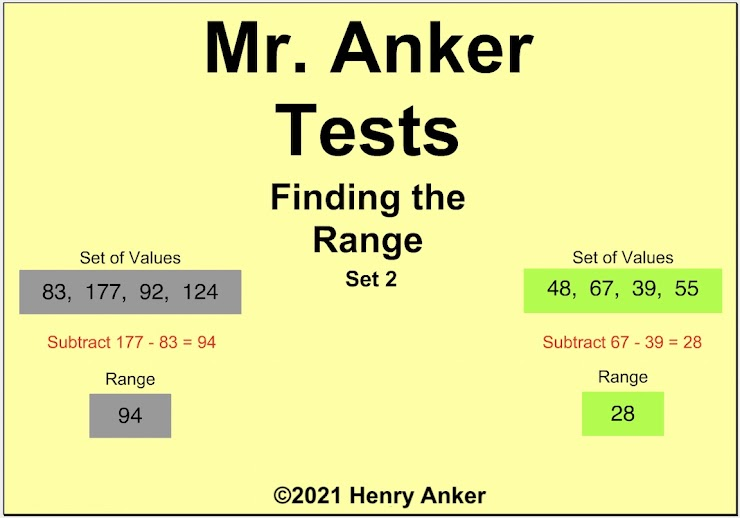Finding the Range Help Slide 1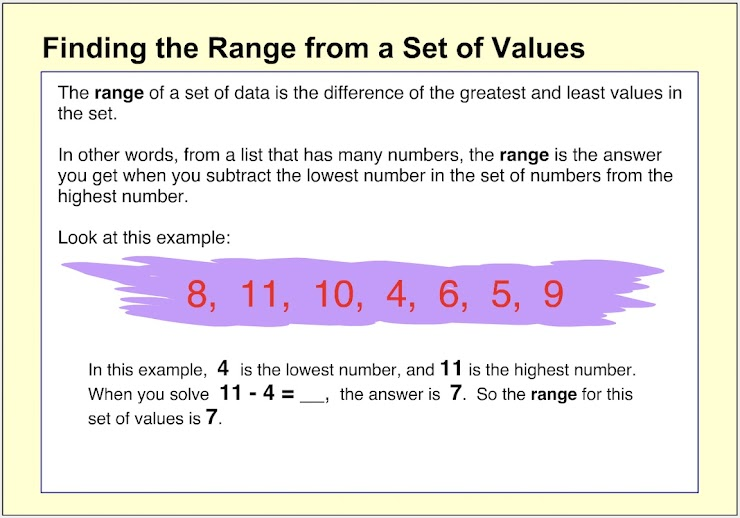Finding the Range Help Slide 2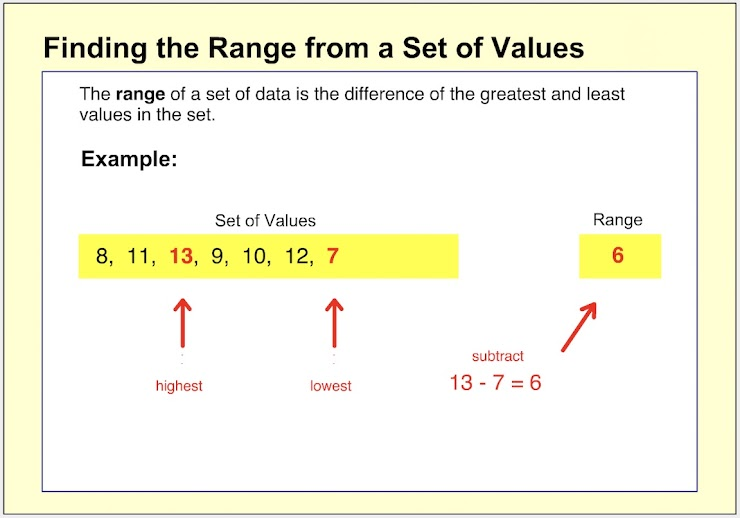Finding the Range Help Slide 3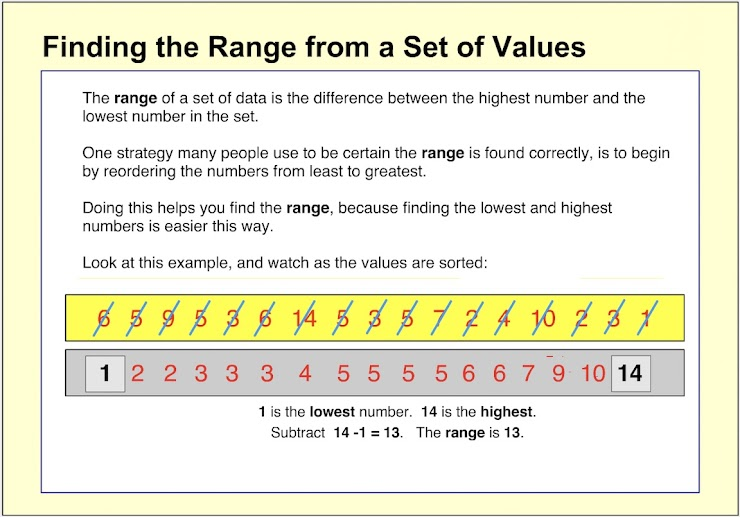Finding the Range Help Slide 4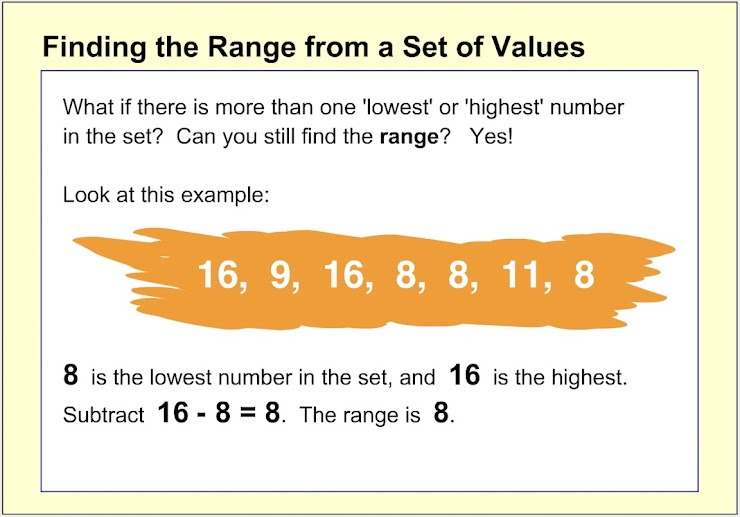Finding the Range Help Slide 5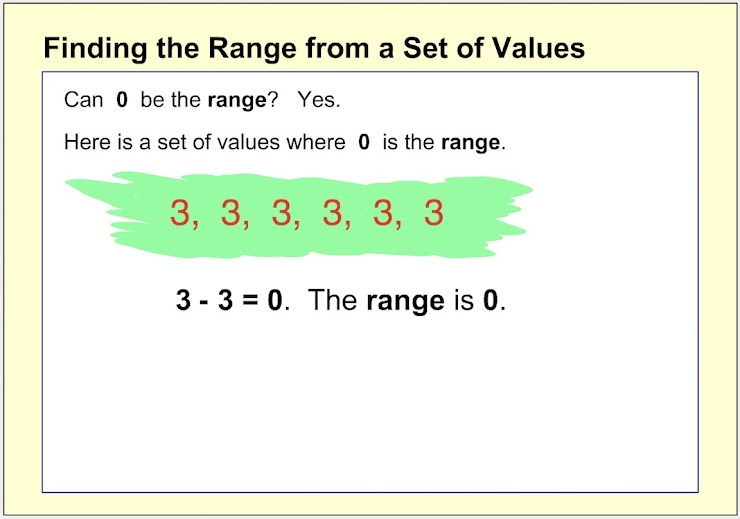Finding the Range Help Slide 6 (more advanced...)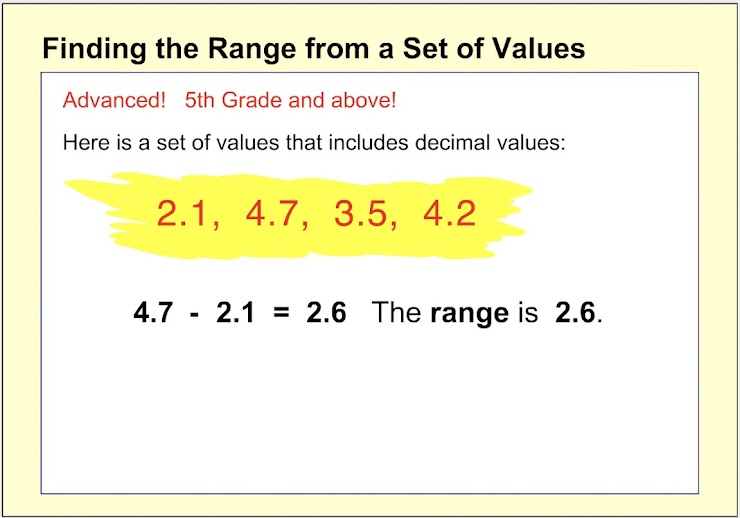Finding the Range Help Slide 7 (more advanced...)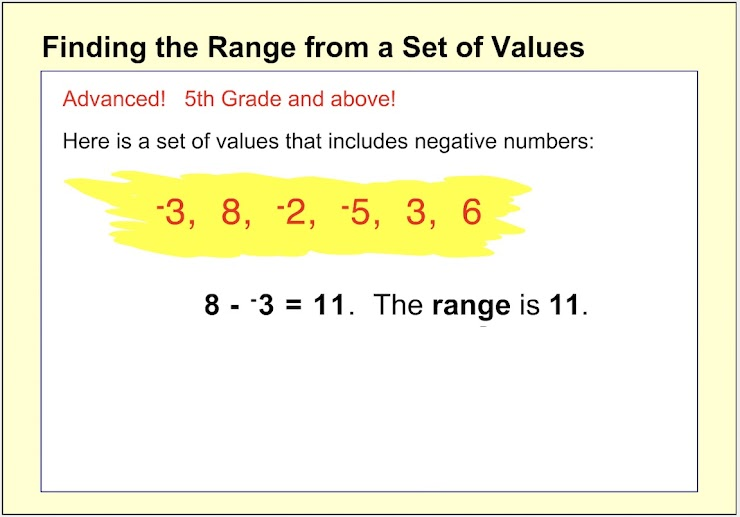1.  Which choice below is the range for the set of values shown? *
1 point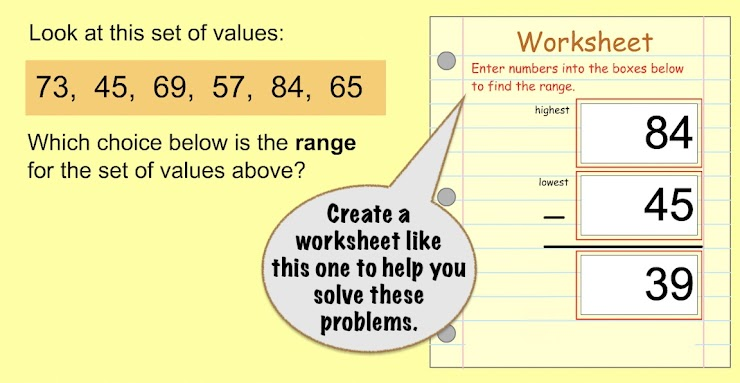2.  Which choice below is the range for the set of values shown? *
1 point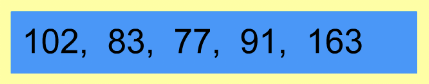Remember to use a worksheet like the one on the first question to help you solve this one and the rest.
3. Which choice below is the range for the set of values shown? *
1 point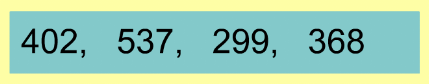4. Which choice below shows the correct equation to use to find the range? *
1 point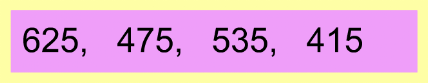5.  On the line below, enter the range for the set of values shown. *
1 point6.  On the line below, enter the range for the set of values shown. *
1 point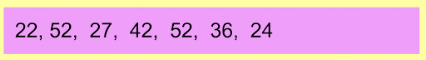7.  On the line below, enter the range for the set of values shown. *
1 point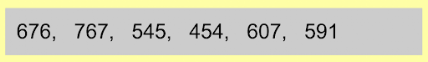8.  On the line below, enter the range for the set of values shown. *
1 point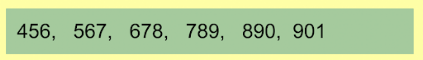9.  On the line below, enter the range for the set of values shown. *
1 point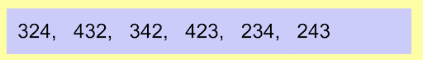10.  On the line below, enter the range for the set of values shown. *
1 point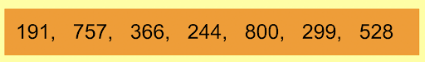Submit
Clear form